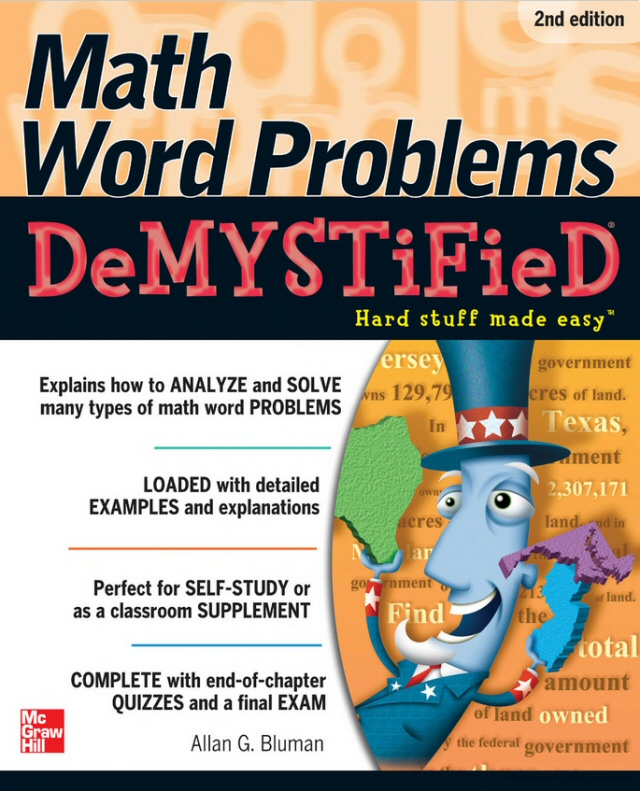# Math Word Problems Demystified

####Your solution to MATH word PROBLEMS!

by Allan Bluman, 2nd edition (2011)

Find yourself stuck on the tracks when two trains are traveling at different speeds? Help has arrived! Math Word Problems Demystified, Second Edition is your ticket to problem-solving success.

Based on mathematician George Polya's proven four-step process, this practical guide helps you master the basic procedures and develop a plan of action you can use to solve many different types of word problems. Tips for using systems of equations and quadratic equations are included. Detailed examples and concise explanations make it easy to understand the material, and end-of-chapter quizzes and a final exam help reinforce learning.

It's a no-brainer! You'll learn to solve:

• Decimal, fraction, and percent problems
• Proportion and formula problems
• Number and digit problems
• Distance and mixture problems
• Finance, lever, and work problems
• Geometry, probability, and statistics problems

Simple enough for a beginner, but challenging enough for an advanced student, Math Word Problems Demystified, Second Edition helps you master this essential mathematics skill.

Preis:
EUR 23,95
Versandfertig: sofort
Artikel wird weltweit versandkostenfrei geliefert.